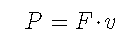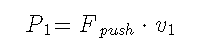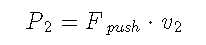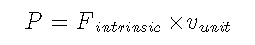# Power and its intrinsic force

Keying Guan

Department of Mathematics, School of Science, Beijing Jiaotong University

Emailkeying.guan@gmail.com

Almost all textbooks of physics or mechanics give the basic relationship between power P and force F（1）

But in fact, the mainstream academic community has not yet formed a clear consensus on how to correctly interpret this formula, especially the interpretation speed in the formula. Specifically, in addition to the difference in the understanding of formula (1) between Einstein and the mainstream theory on the light pressure problem (https://blog.sciencenet.cn/home.php?mod=space&uid=553379&do=blog&id=1382875) ,  it is also specifically manifested in various understandings of formula (1).

For example, today I searched for "the relationship between power and force" in Chinese, and I got the following explanations in turn:

1) The formula for calculating power is power = work/time. Power represents the physical quantity of how fast or slow the work is done. The work done per unit time is called power, denoted by P. Therefore, the power is equal to the scalar product of the applied force and the velocity of the point where the force is applied.

(From Baidu Encyclopedia)

2) What is the relationship between power, force and speed?

Feb 20, 2019 — Power is the product of force and velocity, with the formula: P=FV. The relationship between power and force: When the speed is constant, the power is proportional to the force. The relationship between power and speed: When the force is constant, the power is proportional to the speed.

(from baidu.com https://zhidao.baidu.com › question)

3) What is the relationship between power and speed

Oct 3, 2020 —The relationship between power and force: When the speed is constant, the power is proportional to the force. When the force is in the same direction as the motion of the object, the power of the force doing work is P=Fv. When it is the average speed, P means the average power, when it is the ...

(from chusan.com http://www.chusan.com › zhongkao)

4) Interpretation of high school physics knowledge points: calculation of work and power_mechanical energy

Jan 7, 2021 — Calculation of work plays a very important role in middle school physics. The formula W=FScosa of work learned in middle school can only be used in the case of constant force work, and there is no fixed formula for the calculation of variable force work available, ...

(from sohu.com http://www.sohu.com › ...)

etc.

When I Googled "the relationship between power and force" in English, I first saw the following explanation:

power = force × distance (in direction of the force) time taken, so power = force × velocity. (However, this only works if the velocity is steady, i.e. the force is not the resultant force on the moving object.)

(from: Episode 218: Mechanical power | IOPSpark - Institute of Physics,

https://spark.iop.org › episode-218-mechanical-power)

Undoubtedly, many of the above explanations are correct under limited conditions, but they also expose a common problem worth pondering. The problem is explained below:

The force F in equation (1) is clearly the force that pushes the object in motion, more accurately denoted Fpush. When the velocity of the pushed object becomes stable, the stable condition should be that the driving force is equal to the motion resistance experienced by the pushed object.  In general, the movement resistance is positively correlated with the movement speed of the object, that is, the greater the movement speed, the greater the movement resistance encountered (not necessarily proportional). Therefore, in different environments (such as different friction coefficients), when the same object receives the same large motion resistance, the speed exhibited by the object must also be different. Therefore, under different environments, under the action of a given thrust Fpush, the speed at which the object stabilizes will also be different. At this point, is formula (1) correct? That is to say, in different environments, in order to achieve the same driving force, does the force exerter (human, animal, or engine) really need to output (according to formula (1)) different power?

Take a cyclist, for example. Assuming that the bicycle is equipped with a speed change system, when driving on a flat road, the cyclist will generally step on the bicycle pedal with the average force Fpush that he feels comfortable with, driving himself and the bicycle to move forward at a satisfactory speed v1. At this time, if the average power P1 consumed by the rider is calculated according to formula (1), we get(2)

When a strong headwind is encountered during riding, resulting in greater air resistance, the rider hopes to pedal with the force Fpush that feels comfortable to him. To do this, he can keep himself and the bike going at a slower average speed v2 through the gear change. At this time, according to formula (1), the power consumed by the rider should be as small as(3)

But people's actual feeling is that in the same long period of time, the energy (or average power) consumed by the cyclist under the two conditions (expressed in the fatigue felt by the cyclist) is similar. That is to say, the power calculated according to the formula (3) is not really paid by the rider.

This question can also be put another way:

Study a car driving on a flat road. In the case of safety, for the sake of convenience, when the driving speed of the car stabilizes at a satisfactory constant speed v1, the driver will keep the accelerator (fuel supply speed) and the car gear unchanged, the effective power P1 consumed by the car It is related to the corresponding thrust Fpush and satisfies formula (1). When the car encounters a large resistance caused by the headwind, if the driver still keeps the accelerator and the car gear in the original state, it is reasonable to think that the effective power P consumed by the car and the corresponding thrust Fpush are basically the same as before the headwind. However, due to the increased resistance, the vehicle speed will automatically slow down until the thrust Fpush is balanced with the new total resistance, and the vehicle speed stabilizes at a new value v2 which is significantly smaller than v1. After reaching the new speed, if the average power P2 of the vehicle is calculated according to formula (3), the obtained value must be less than P1. This means that when encountering greater wind resistance, if the driver maintains the original accelerator (fuel supply speed) and the gear of the car, the power of the car engine will be reduced. Clearly, this is at odds with maintaining real control over fueling speed and gear.

The above examples illustrate the important issues left by mainstream explanations that need to be studied in depth.

In fact, although power and force are two different physical concepts, there is neither force generated by power nor power independent of force in the world, and they must appear simultaneously in the process of material interaction. In the actual interaction of two objects, one party is often the active producer of power and force in the interaction, which can be called the force exerter. When the force exerter pays a stable power P, it will inevitably produce a corresponding stable thrust Fpush, but the steady speed v of the force recipient may be different, which is caused by the different resistance environments of the force-bearing object. This fact shows that the formula (3 ) cannot simply be used to describe the intrinsic relationship between power P and thrust Fpush. Especially when the subject is in a static state before receiving a given force, the velocity of the subject must be zero at the moment when the force begins to act, and the relationship between force and force at this time cannot be determined by the expression of formula (3) .

However, no matter what the conditions, power and force always occur naturally together. This fact shows that the power P naturally contains such a force F, which is not affected by the internal structure of the generator, nor the external environment, and it only depends on the power P itself. This article refers to this force as the intrinsic force of power and expresses it as Fintrinsic.

In fact, based on the existing mechanical definitions, formulas and dimensional analysis, and based on the principle of formulating the unit system, the author has theoretically confirmed that any given power has its intrinsic force. The relationship between the power and its intrinsic force is reflected by the formula (18) in the blog post

With the current notation, the formula can be expressed precisely as(4)

where vunit is the directionless unit velocity in the chosen system of units, and the power P and its intrinsic force Fintrinsic in the formula should be measured in the same chosen system of units. If the International System of Units (SI) is used, the unit of speed is one meter per second, power P must be measured in Watts (Watt, or W), and intrinsic power Fintrinsic must be measured in Newtons (Newton, or N). The unit speed in the formula has nothing to do with the speed displayed by the object under force.

It is not difficult to see that in any complete system of units, P and Fintinsic have the same value after subtracting the dimensions. Therefore, the definition of Fintrinsic here is reasonable and natural.

In addition, the author has discussed the direction issues involved in power P and intrinsic force Fintrinsic in previous blog posts. Interested readers can refer to this paper (refer to Rediscussing on the Force-Power equivalence ). According to relevant discussions, the author believes that the unit velocity vunit in formula (4) is just a scalar, and the product in the formula is not a scalar product, but a common product.

It is worth noting that: as mentioned above: "especially when the force-bearing device is in a static state before the force is applied, the speed of the force-bearing device must be zero at the moment of force,...", obviously, the force at this time can be Considering it to be instantaneous static force, formula (4) still exists objectively. If the static resistance received by the receiver is greater than the given thrust, the object will remain stationary. If the forcer continues to send out the same force and apply the same force, the object will continue to be at rest, because the static resistance is too large, so this thrust has just become a real static force. At this time, both the donor and the acceptor are subjected to thrust and reaction forces, and corresponding internal changes occur, such as heating, structural changes or damage. These changes in the receiver are obviously a manifestation of the energy provided by the giver. Because of this, the author came to the conclusion that "static force does do work". This conclusion negates the misconception that the mainstream theory holds that "static force does not do work". These misconceptions ignore the energy expended by a weightlifter lifting a barbell and holding it still, and by a tug-of-war athlete leveling a deadlock with an opponent in a tug-of-war contest. In addition, the mainstream theory also ignores the microscopic changes and damage inside the object under the action of static force.

There are a lot of static phenomena in nature, so it is of great significance to study the corresponding internal changes of matter under the action of static force. The conclusion and formula (4) that the static force also does work will definitely help the research in this area (including quantitative research). This is what the mainstream theory should pay attention to.

https://blog.sciencenet.cn/blog-553379-1384139.html

## 全部精选博文导读

GMT+8, 2023-10-3 01:33